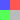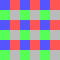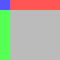Defines a bitmap fill. The bitmap can be smoothed, repeated or tiled to fill the area; or manipulated using a transformation matrix. Use a GraphicsBitmapFill object with the `Graphics.drawGraphicsData()` method. Drawing a GraphicsBitmapFill object is the equivalent of calling the `Graphics.beginBitmapFill()` method.

### `new (?bitmapData:BitmapData, ?matrix:Matrix, repeat:Bool = true, smooth:Bool = false)`

Creates a new GraphicsBitmapFill object.

Parameters:

`bitmapData` A transparent or opaque bitmap image that contains the bits to display. A matrix object (of the openfl.geom.Matrix class), which you use to define transformations on the bitmap. If `true`, the bitmap image repeats in a tiled pattern. If `false`, the bitmap image does not repeat, and the edges of the bitmap are used for any fill area that extends beyond the bitmap. If `false`, upscaled bitmap images are rendered using a nearest-neighbor algorithm and appear pixelated. If `true`, upscaled bitmap images are rendered using a bilinear algorithm. Rendering that uses the nearest-neighbor algorithm is usually faster.

### `bitmapData:BitmapData`

A transparent or opaque bitmap image.

### `matrix:Matrix`

A matrix object (of the openfl.geom.Matrix class) that defines transformations on the bitmap. For example, the following matrix rotates a bitmap by 45 degrees (pi/4 radians):

``````var matrix = new openfl.geom.Matrix();
matrix.rotate(Math.PI / 4);``````

### `repeat:Bool`

Specifies whether to repeat the bitmap image in a tiled pattern. If `true`, the bitmap image repeats in a tiled pattern. If `false`, the bitmap image does not repeat, and the outermost pixels along the edges of the bitmap are used for any fill area that extends beyond the bounds of the bitmap.

For example, consider the following bitmap (a 20 x 20-pixel checkerboard pattern):When `repeat` is set to `true` (as in the following example), the bitmap fill repeats the bitmap:When `repeat` is set to `false`, the bitmap fill uses the edge pixels for the fill area outside the bitmap:### `smooth:Bool`

Specifies whether to apply a smoothing algorithm to the bitmap image. If `false`, upscaled bitmap images are rendered by using a nearest-neighbor algorithm and look pixelated. If `true`, upscaled bitmap images are rendered by using a bilinear algorithm. Rendering by using the nearest neighbor algorithm is usually faster.